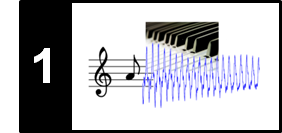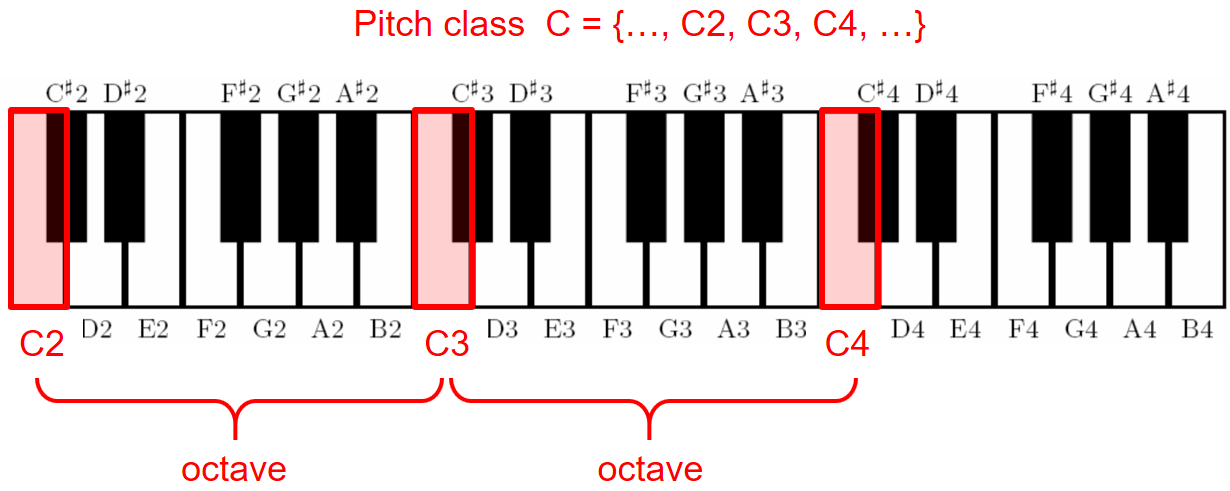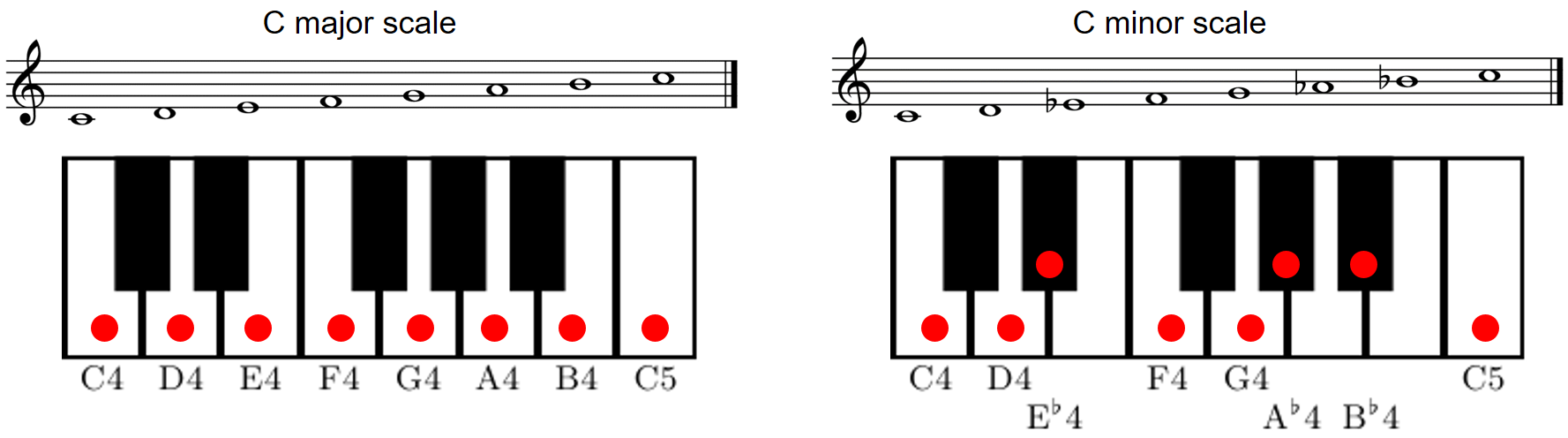# Musical Notes and Pitches

Following Section 1.1.1 of [Müller, FMP, Springer 2015], we introduce in this notebook the notions of musical notes, pitches, and the twelve-tone equal-tempered scale.

## Notes and Pitches¶

In music, the term note is often used in a rather loose way and may refer to both a musical symbol (when talking about score representations) as well as a pitched sound (when talking about audio representations). When thought of a musical symbol, each note has several attributes that determine the relative duration and the pitch of a sound to be performed by a musician. The notion of pitch is not strict and refers to a perceptual property that allows a listener to order a sound on a frequency-related scale. As discussed in Section 1.3 of [Müller, FMP, Springer 2015], playing a note on an instrument results in a (more or less) periodic sound of a certain fundamental frequency. This fundamental frequency is closely related to what is meant by the pitch of a note. The term "pitch" allows us to order pitched sounds from "lower" to "higher"—similarly to the keys of a piano keyboard ordered from left to right.

## Pitch Class and Octaves¶

Two notes with fundamental frequencies in a ratio equal to any power of two (e.g., half, twice, or four times) are perceived as very similar. Because of that, all notes with this kind of relation can be grouped under the same pitch class. This observation also leads to the fundamental notion of an octave, which is defined to be the interval between one musical note and another one with half or double its fundamental frequency. Using this definition, a pitch class is a set of all pitches or notes that are an integer number of octaves apart. The following figure and sound example illustrates these concepts for the pitch class $\mathrm{C}$:In :
import numpy as np
import IPython.display as ipd

def generate_sinusoid_pitches(pitches=, dur=0.5, Fs=4000, amp=1):
"""Generation of sinusoids for a given list of MIDI pitches

Notebook: C1/C1S1_MusicalNotesPitches.ipynb

Args:
pitches (list): List of MIDI pitches (Default value = )
dur (float): Duration (in seconds) of each sinusoid (Default value = 0.5)
Fs (scalar): Sampling rate (Default value = 4000)
amp (float): Amplitude of generated signal (Default value = 1)

Returns:
x (np.ndarray): Signal
t (np.ndarray): Time axis (in seconds)
"""
N = int(dur * Fs)
t = np.arange(N) / Fs
x = []
for p in pitches:
freq = 2 ** ((p - 69) / 12) * 440
x = np.append(x, np.sin(2 * np.pi * freq * t))
x = amp * x / np.max(x)
return x, t

dur = 1
Fs = 22050

pitches = [36,48,60,72,84,96,108]
x, t = generate_sinusoid_pitches(pitches=pitches, dur=dur, Fs=Fs, amp=0.5)
print('Pitch class C = {..., C1, C2, C3, C4, C5, C6, C7, ...}', flush=True)
ipd.display(ipd.Audio(data=x, rate=Fs))

Pitch class C = {..., C1, C2, C3, C4, C5, C6, C7, ...}


## Musical Scales¶

In order to describe music using a finite number of symbols, one needs to discretize the space of all possible pitches. This leads to the notion of a musical scale, which can be thought of as a finite set of representative pitches. Because of the close octave relationship of pitches, scales are generally considered to span a single octave, with higher or lower octaves simply repeating the pattern. A musical scale can then be specified by a division of the octave space into a certain number of scale steps. The elements of a scale are often simply referred to as the notes of the scale and are ordered according to their respective pitches. The following figure illustrates the case of a C major scale and a (natural) C minor scale.In :
dur = 0.5
Fs = 22050

x_maj, t = generate_sinusoid_pitches(pitches=[60,62,64,65,67,69,71,72], dur=dur, Fs=Fs, amp=0.5)
x_min, t = generate_sinusoid_pitches(pitches=[60,62,63,65,67,68,70,72], dur=dur, Fs=Fs, amp=0.5)

print('C major scale', flush=True)
ipd.display(ipd.Audio(data=x_maj, rate=Fs))
print('C minor scale', flush=True)
ipd.display(ipd.Audio(data=x_min, rate=Fs))

C major scale

C minor scale